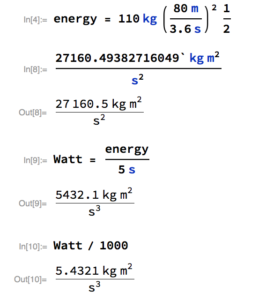# EMAk v1

So I’ve been wanting to make a Go-kart for like…well since Mario kart probably. By the way, the guy in that link just wrecks it….Anyways, so while deciding a new project, I had the choice between a laser cutter and the gokart.

I guess you’ve figured out already what I decided to build.

So, like the last project, a person should write down the kind of things they want to do, or rather, what they want the machine to do, So here it goes:

1. Go fast
2. ….

Ok. maybe thats a little short. How about we think of some limitations I’ll artificially put on the machine

1. It should be small. Almost uncomfortably
2. It should be fast, no less than 60 Km/h
3. Under 1000 euros
4. others

Well, ok that’s not so bad. There some other things I’d like to throw in there, A power governer, one that a person actually has to manually switch in order to go to super speed mode (60km/h +), an LCD speedometre, and maybe a battery charger display.

A friend of mine and I talked about it to today just to see approximately what a person would need for motor size (in KW),

We can use the Energy conservation law and namely the right side of it;

$T_1+U_1 = T_2+U_2$

$T=\frac{1}{2}mv^{2}+ mg\Delta\mathrm{x}$ Delta X in height above the ground. Which is in our idealized system 0.

I weigh about ahmmmm…80Kg and the machine itself will weigh a max of 30Kg (we wanna go fast) so in total 110Kg

I wanna go 80Km/h for max speed.

So we get

$T=\frac{110Kg}{2} \times (\frac{80000\mathrm{m}}{3600\mathrm{s}})^{2}$ Which is equal to about 27 KJ. I want to get to about 80km/h within 5 seconds. So to find out how many Watts we need, we can use the Power equation:

Approx Watts required: $P = \frac{T}{t}\approx\frac{27KJ}{5\mathrm{s}}\approx5.4KW$

So people can see what happened;Alright. So in an ideal frictionless world. I’m looking for either a single motor at 5.4KW, or two motors half of that each (which I’ll ideally be doing)

Thats a nice start.

$e^{\sqrt{2}}$

[/latex]